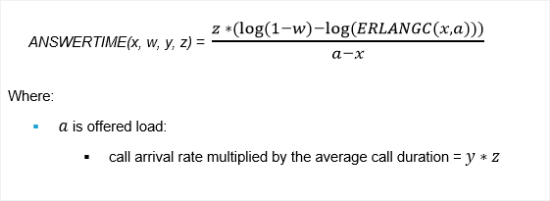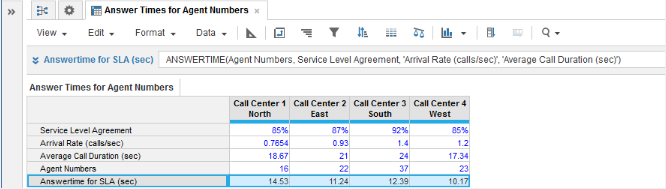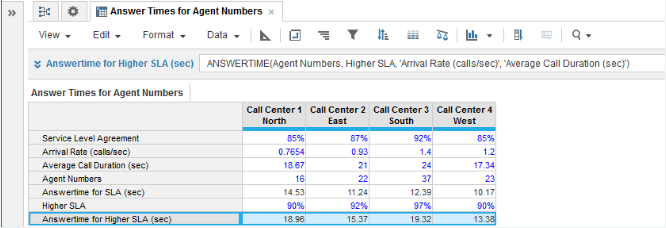1. Calculation functions
2. All Functions
3. Call Center Planning FunctionsCalculates the minimum waiting time required to maintain the service level agreement.

## Syntax

ANSWERTIME(x, w, y, z)

where:

• x: is the number of servers, telephone lines, or agents
• w: service level agreement or the percentage of calls to be answered within the target answer time
• y: arrival rate of calls or the number of calls per unit of time
• z: average call duration

## Format

Input Format Output Format

x: number

w: number (percentage)

y: number (time unit)

z: number (time unit)

Number

## Arguments

The function uses the following arguments:

• x: Numeric line item, property, or expression
• w: Numeric line item, property, or expression
• y: Numeric line item, property, or expression - must be the same time unit as used for argument z
• z: Numeric line item, property, or expression - must be the same time unit as used for argument y

## Constraints

The function has the following constraints:

• All parameters are mandatory.
• All parameters must be a numeric line item, property, or expression.
• Time unit used for y and z parameters must be the same.
• Result line item must be numeric.
• Maximum number of agents is five million.

ANSWERTIME is the solution to this equation:## Excel equivalent

• No Excel equivalent

## Examples

### Example 1

In this example, we have four call centers each covering a separate geographical area:

• Each call center must achieve a service level agreement (SLA), expressed as the percentage of incoming calls that will be answered for a given target answer time.
• The agent staffing levels for each call center are known.
• The arrival rate of incoming calls and the average call duration are known for each call center from historical monitoring of operational performance.

With the baseline metrics entered for each call center in numeric source line items for Service Level Agreement, Arrival Rate (calls/sec), Average Call Duration, and Agent Numbers, we can use ANSWERTIME in a numeric result line item to calculate the minimum waiting time for incoming calls at each call center while maintaining the SLA:### Example 2

A second example extends Example 1 to perform what-if analysis for minimum call waiting times at call centers. Suppose you want to know what will be the minimum call waiting times for each call center, if a higher SLA is maintained. The Higher SLA numeric source line item allows you to enter higher percentages for the SLA. The Answertime for Higher SLA (sec) numeric result line item then provides the minimum call waiting times for the increased SLAs: